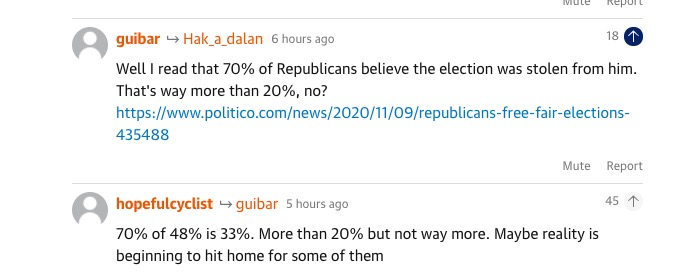# What is the difference between 33% and 20%?

“way more” or not “way more”? That is the question.https://www.theguardian.com/commentisfree/2020/nov/13/trump-coup-virtual-confederacy-race-legal-trumpian

If you’ve read anything I’ve written in Towards Data Science, you’ll know that taking differences and ratios of probabilities is utter nonsense.

Of course, nothing prevents you from rebutting the argument in the second comment in the above image by saying, “33% vs. 20% is a 65% increase because 65% = (33–20)/20! That is yuuuge!”

Nothing prevents you from saying that you can multiply two complex numbers (in their tuple representation) by

(a,b) * (c,d) = (a*c, b*d).

You could, but you would never do that, would you?

For the same underlying reasons you wouldn’t do that, you can’t take the difference of probabilities.

But there is an algebra of probabilities. The way to compare two probabilities (for the difference in probabilities for the same outcome given different conditions) is to take the difference in the log of the odds, or to take the ratio of the odds, which is the relative odds ratio. (You can derive that from Bayes’ Theorem, a first year probability exercise, so don’t take my word for it.)

So, for the above example, what you really have is that the odds doubled (of belief that the election was stolen from Him, who shall not be named):

relative odds ratio = (33/(100–33)) / ((100–80)/20) = 2

Please think about it before you turn your brains off and start analysing A/B test results or doing Measurement and Evaluation.

“But we have AI, so why do we need our brains?”

(Deflated sigh: “I don’t know.”)

## More from Ranjeet Tate

I stop to miau to cats.